U.S. Department of Transportation
1200 New Jersey Avenue, SE
Washington, DC 20590
202-366-4000

Federal Highway Administration Research and Technology
Coordinating, Developing, and Delivering Highway Transportation InnovationsThis report is an archived publication and may contain dated technical, contact, and link information
 Federal Highway Administration > Publications > Research Publications > 03060 > Appb.Cfm Concrete Mixture Optimization Using Statistical Methods
 Publication Number: FHWA-RD-03-060

# APPENDIX B. Experiment Design and Data Analysis for Factorial Experiment

## B.1 Experiment Design and Response Data

Table B-1. Factorial experiment: design by volume fraction of factors

Std Order Run Order Point Factor Aw/c Factor B Fine Agg Factor C Coarse Agg Factor DHRWRA Factor E Silica Fume
17 1 Center 0.39525 0.2712 0.4212 0.0060 0.0200
11 2 Fact 0.3576 0.2853 0.4071 0.0069 0.0247
7 3 Fact 0.3576 0.2853 0.4353 0.0051 0.0247
10 4 Fact 0.4329 0.2571 0.4071 0.0069 0.0247
4 5 Fact 0.4329 0.2853 0.4071 0.0051 0.0247
12 6 Fact 0.4329 0.2853 0.4071 0.0069 0.0153
2 7 Fact 0.4329 0.2571 0.4071 0.0051 0.0153
1 8 Fact 0.3576 0.2571 0.4071 0.0051 0.0247
18 9 Center 0.39525 0.2712 0.4212 0.0060 0.0200
8 10 Fact 0.4329 0.2853 0.4353 0.0051 0.0153
9 11 Fact 0.3576 0.2571 0.4071 0.0069 0.0153
6 12 Fact 0.4329 0.2571 0.4353 0.0051 0.0247
3 13 Fact 0.3576 0.2853 0.4071 0.0051 0.0153
14 14 Fact 0.4329 0.2571 0.4353 0.0069 0.0153
15 15 Fact 0.3576 0.2853 0.4353 0.0069 0.0153
13 16 Fact 0.3576 0.2571 0.4353 0.0069 0.0247
19 17 Center 0.39525 0.2712 0.4212 0.0060 0.0200
5 18 Fact 0.3576 0.2571 0.4353 0.0051 0.0153
16 19 Fact 0.4329 0.2853 0.4353 0.0069 0.0247
25 20 Axial 0.39525 0.2712 0.4494 0.0060 0.0200
21 21 Axial 0.47055 0.2712 0.4212 0.0060 0.0200
23 22 Axial 0.39525 0.2994 0.4212 0.0060 0.0200
27 23 Axial 0.39525 0.2712 0.4212 0.0078 0.0200
20 24 Axial 0.31995 0.2712 0.4212 0.0060 0.0200
31 25 Center 0.39525 0.2712 0.4212 0.0060 0.0200
26 26 Axial 0.39525 0.2712 0.4212 0.0042 0.0200
28 27 Axial 0.39525 0.2712 0.4212 0.0060 0.0106
24 28 Axial 0.39525 0.2712 0.3930 0.0060 0.0200
29 29 Axial 0.39525 0.2712 0.4212 0.0060 0.0294
22 30 Axial 0.39525 0.243 0.4212 0.0060 0.0200
30 31 Center 0.39525 0.2712 0.4212 0.0060 0.0200

Table B-2. Factorial experiment: slump and 1-day strength data

Run Point Slump (mm) 1-Day Strength (MPa)
1Center767016.616.016.2
2Fact444422.222.523.0
3Fact131322.517.922.0
4Fact10210215.816.716.8
5Fact575716.416.716.1
6Fact14014613.414.213.2
7Fact706413.911.113.7
8Fact131317.722.820.5
9Center898317.918.618.7
10Fact10210215.215.215.2
11Fact14014020.920.720.4
12Fact323213.818.818.9
13Fact131324.523.324.7
14Fact767617.317.217.2
15Fact131322.719.521.8
16Fact131320.820.621.4
17Center516419.418.918.1
18Fact322521.422.222.1
19Fact383216.016.116.3
20Axial383818.719.318.9
21Axial12111414.514.914.0
22Axial706418.017.817.5
23Axial646419.320.020.4
24Axial191326.025.427.7
25Center837620.217.319.6
26Axial646418.919.518.9
27Axial15215216.417.016.9
28Axial959520.120.819.7
29Axial383217.220.118.0
30Axial10210217.717.017.7
31Center767616.819.618.8

Table B-3. Factorial experiment: 28-day strength and RCT charge passed data

Run Point 28-Day Strength (MPa) RCT Charge Passed (coulombs)
1 Center 54.0 63.0 - - 263 296 300
2 Fact 59.4 60.2 - - 186 472 268
3 Fact 53.5 52.8 51.7 - 151 151 178
4 Fact 62.7 60.8 55.1 62.9 280 329 279
5 Fact 52.6 56.6 55.8 - 273 236 262
6 Fact 60.4 52.1 60.6 61.3 553 585 485
7 Fact 50.1 49.4 51.5 - 468 550 490
8 Fact 51.0 56.3 47.2 55.2 253 240 208
9 Center 63.5 62.5 - - 247 315 305
10 Fact 53.8 54.2 56.3 - 460 437 439
11 Fact 61.6 64.5 60.8 - 415 427 393
12 Fact 56.5 55.3 56.8 - 258 258 240
13 Fact 58.1 50.2 54.4 - 343 362 317
14 Fact 52.3 46.6 52.1 - 596 527 481
15 Fact 60.3 58.7 58.6 - 218 288 330
16 Fact 60.2 58.5 62.9 - 208 194 216
17 Center 59.9 54.5 55.5 - 299 327 318
18 Fact 58.4 60.1 56.3 - 360 364 340
19 Fact 65.0 59.9 63.9 - 242 243 206
20 Axial 56.7 57.0 62.1 - 168 242 224
21 Axial 53.9 51.1 56.7 - 463 461 449
22 Axial 60.7 62.9 63.6 - 319 305 257
23 Axial 68.4 66.8 67.1 - 272 280 251
24 Axial 62.1 59.4 54.0 - 190 184 192
25 Center 58.2 55.8 56.8 - 239 246 287
26 Axial 52.9 48.2 51.6 - 258 281 280
27 Axial 51.3 55.2 56.8 - 704 766 644
28 Axial 50.0 55.2 54.8 - 268 302 351
29 Axial 55.5 53.8 56.2 - 163 170 153
30 Axial 50.4 49.0 51.6 - 340 304 351
31 Center 55.8 53.3 56.5 - 262 294 274

## B.2.1 Slump

Table B-4. Factorial experiment: sequential model sum of squares for slump

Source Sum of Squares DF Mean Square F Value Prob > F
Mean130296.61130296.6--
Linear 31972.3856394.489.10< 0.0001
2FI 10539.29101053.932.250.0755
Cubic (aliased)2316.865463.371.100.4593
Residual2104.405420.88--
Total 179828.3315800.91--

Table B-5. Factorial experiment: lack-of-fit test for slump

Source Sum of Squares DF Mean Square F Value Prob > F
Linear 17103.6121814.467.150.0345
2FI 6564.3211596.765.240.0618
Cubic (aliased) 1648.7511648.7514.470.0190
Pure error 455.644113.91--

Table B-6. Factorial experiment: ANOVA for slump model

Source Sum of Squares DF Mean Square F Value Prob > F
Model 40109.97 7 5730.00 13.99 < 0.0001
A 12138.75 1 12138.75 29.63 < 0.0001
B 606.52 1 606.52 1.48 0.2360
C 6048.38 1 6048.38 14.77 0.0008
D 2426.07 1 2426.07 5.92 0.0231
E 10752.67 1 10752.67 26.25 < 0.0001
AB 1837.19 1 1837.19 4.48 0.0452
CD 6300.39 1 6300.39 15.38 0.0007
Residual 9421.67 23 409.64 - -
Lack of fit 8966.03 19 471.90 4.14 0.0887
Pure error 455.64 4 113.91 - -
Cor total 49531.64 30 - - -

Table B-7. Factorial experiment: summary statistics for slump model

 Std. Dev. Mean C.V. 20.24 R-Squared 0.8098 64.83 Adj R-Squared 0.7519 31.22 Pred R-Squared 0.6275 18452.2 Adeq Precision 15.62

Table B-8. Factorial experiment: coefficient estimates for slump model

Factor Coefficient Estimate DF Standard Error 95% CI Low 95% CI High
Intercept64.8313.6457.3172.35
A (w/c)22.4914.1313.9431.04
B (fine agg)-5.0314.13-13.573.52
C (coarse agg)-15.8714.13-24.42-7.33
D (HRWRA)10.0514.131.5118.60
E (silica fume)-21.1714.13-29.71-12.62
AB10.7215.060.2521.18
CD-19.8415.06-30.31-9.38

Model equation for slump in terms of coded factors:

Slump = 64.83 + 22.49*A - 5.03*B - 15.87*C + 10.05*D - 21.17*E + 10.72*A*B - 19.84*C*D

Model equation for slump in terms of actual factors:

 Slump = -1365.5 - 4876.9*w/c - 8334.7*fine agg + 8256.5*coarse agg + 6.6982 x 105*HRWRA - 4503.6*silica fume + 20185*w/c*fine agg - 1.564 x 106 *coarse agg*HRWRA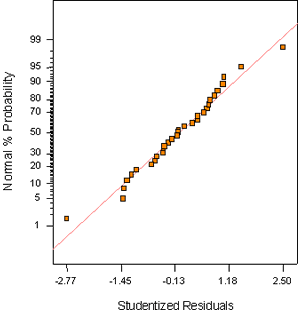Figure B-1. Factorial experiment: normal probability plot for slump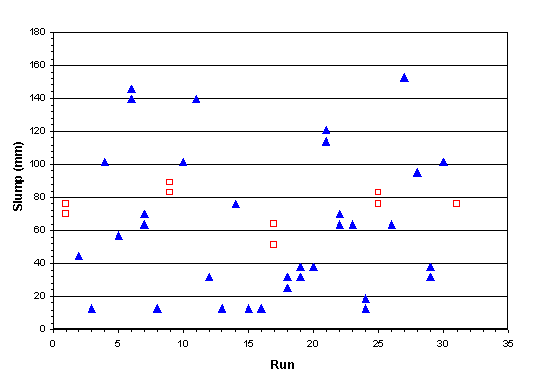Figure B-2. Factorial experiment: raw data plot for slump (hollow squares indicate control runs)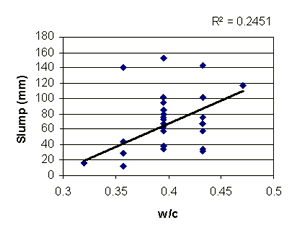Figure B-3. Factorial experiment: scatterplot of slump vs. w/c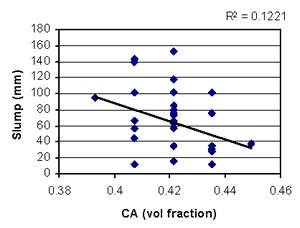Figure B-4. Factorial experiment: scatterplot of slump vs. coarse aggregate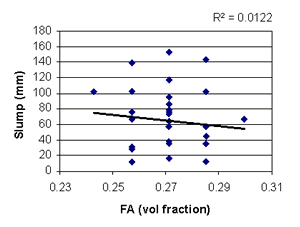Figure B-5. Factorial experiment: scatterplot of slump vs. fine aggregate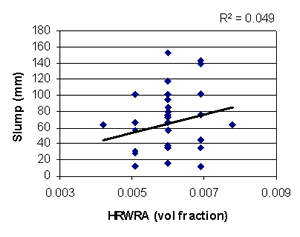Figure B-6. Factorial experiment: scatterplot of slump vs. HRWRA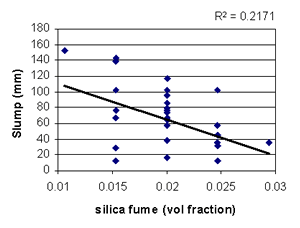Figure B-7. Factorial experiment: scatterplot of slump vs. silica fume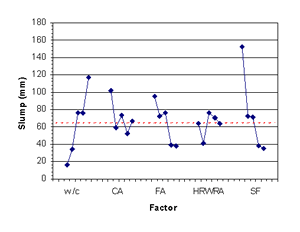Figure B-8. Factorial experiment: means plots for slump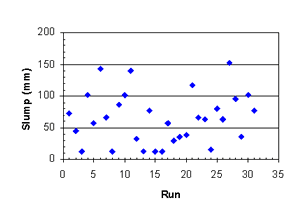Figure B-9. Factorial experiment: slump vs. run sequence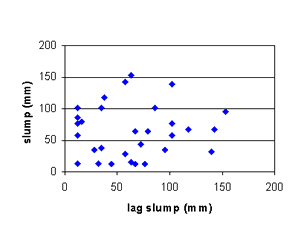Figure B-10. Factorial experiment: lag plot for slump

Table B-9. Factorial experiment: sequential model sum of squares for 1-day strength

Source Sum of Squares DF Mean Square F Value Prob > F
Mean  10780.54 1 10780.54 - -
Linear  215.97 5 43.19 22.15 < 0.0001
2FI 27.35 10 2.73 1.92 0.1236
Quadratic 13.60 5 2.72 3.48 0.0441
Cubic (aliased) 1.86 5 0.37 0.31 0.8865
Residual 5.95 5 1.19 - -
Total  11045.26 31 356.30 - -

Table B-10. Factorial experiment: lack-of-fit test for 1-day strength

Source Sum of Squares DF Mean Square F Value Prob > F
Linear  43.98 21 2.09 1.75 0.3129
2FI  16.63 11 1.51 1.27 0.4444
Quadratic 3.03 6 0.51 0.42 0.8339
Cubic (aliased) 1.18 1 1.18 0.98 0.3772
Pure error 4.78 4 1.19 - -

Table B-11. Factorial experiment: ANOVA for 1-day strength model

Source Sum of Squares DF Mean Square F Value Prob > F
Model 240.87 8 30.11 27.76 < 0.0001
A 213.26 1 213.26 196.66 < 0.0001
B 0.48 1 0.48 0.45 0.5113
C 0.043 1 0.043 0.040 0.8433
E 2.06 1 2.06 1.90 0.1819
A2 6.20 1 6.20 5.72 0.0257
AC 5.15 1 5.15 4.75 0.0404
AE 7.16 1 7.16 6.60 0.0175
BC 6.51 1 6.51 6.00 0.0227
Residual 23.86 22 1.08 - -
Lack of fit 19.08 18 1.06 0.89 0.6248
Pure error 4.78 4 1.19 - -
Cor total 264.72 30 - - -

Table B-12. Factorial experiment: summary statistics for 1-day strength model

 Std. Dev. Mean C.V. 1.04 R-Squared 0.9099 18.65 Adj R-Squared 0.8771 5.58 Pred R-Squared 0.8294 45.16 Adeq Precision 22.583

Table B-13. Factorial experiment: coefficient estimates for 1-day strength model

Factor Coefficient Estimate DF Standard Error 95% CI Low 95% CI High
Intercept 18.29 1 0.24 17.80 18.79
A (w/c) -2.98 1 0.21 -3.42 -2.54
B (fine agg) 0.14 1 0.21 -0.30 0.58
C (coarse agg) 0.043 1 0.21 -0.40 0.48
E (silica fume) 0.29 1 0.21 -0.15 0.73
A2 0.46 1 0.19 0.061 0.86
AC 0.57 1 0.26 0.027 1.11
AE 0.67 1 0.26 0.13 1.21
BC -0.64 1 0.26 -1.18 -0.098

Model equation for 1-day strength in terms of coded factors:

1-day strength = 18.29 - 2.98*A + 0.14*B + 0.043*C + 0.29*E + 0.46*A2 + 0.57*A*C + 0.67*A*E - 0.64*B*C

Model equation for 1-day strength in terms of actual factors:

 1-day strength = -63.8 - 860.8*w/c + 1361.3*fine agg + 450.8*coarse agg - 1431.5*silica fume + 323.9*(w/c)2 + 1068*w/c*coarse agg + 3780*w/c*silica fume - 3208*fine agg*coarse agg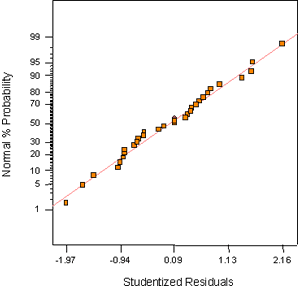Figure B-11. Factorial experiment: normal probability plot for 1-day strength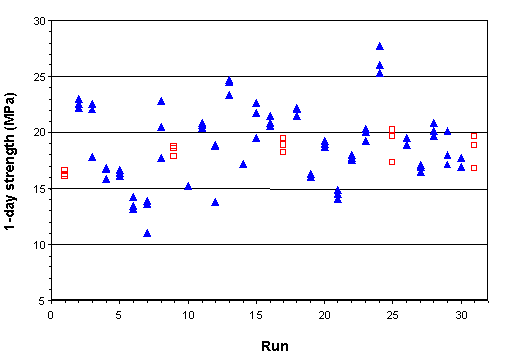Figure B-12. Factorial experiment: raw data plot for 1-day strength (hollow squares indicate control runs)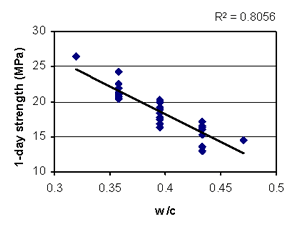Figure B-13. Factorial experiment: scatterplot of 1-day strength vs. w/c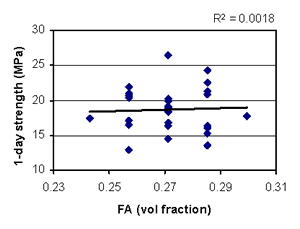Figure B-14. Factorial experiment: scatterplot of 1-day strength vs. fine aggregate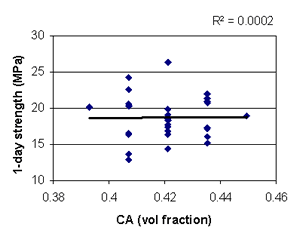Figure B-15. Factorial experiment: scatterplot of 1-day strength vs. coarse aggregate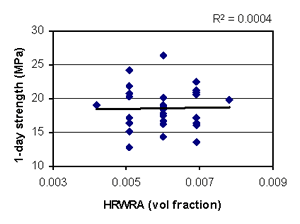Figure B-16. Factorial experiment: scatterplot of 1-day strength vs. HRWRA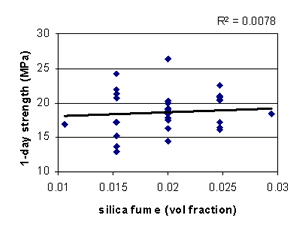Figure B-17. Factorial experiment: scatterplot of 1-day strength vs. silica fume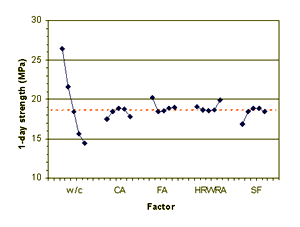Figure B-18. Factorial experiment: means plot for 1-day strength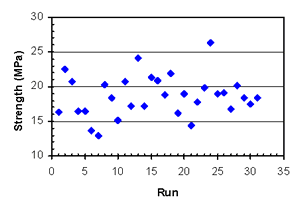Figure B-19. Factorial experiment: 1-day strength vs. run sequence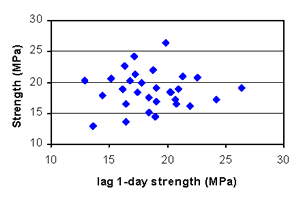Figure B-20. Factorial experiment: lag plot for 1-day strength

## B.2.3. 28-Day Strength

Table B-14. Factorial experiment: sequential model sum of squares for 28-day strength

Source Sum of Squares DF Mean Square F Value Prob > F
Mean 100300.01100300.0--
Linear 292.28558.465.710.0012
2FI 129.241012.921.530.2208
Cubic (aliased) 61.25512.251.620.3050
Residual 37.8457.57--
Total  100848.1313253.17--

Table B-15. Factorial experiment: lack-of-fit test for 28-day strength

Source Sum of Squares DF Mean Square F Value Prob > F
Linear219.79 21 10.47 1.16 0.4972
2FI 90.55 11 8.23 0.91 0.5942
Cubic (aliased)1.76 1 1.76 0.20 0.6813
Pure error36.08 4 9.02 - -

Table B-16. Factorial experiment: ANOVA for 28-day strength model

Source Sum of Squares DF Mean Square F Value Prob > F
Model300.21 475.057.870.0003
A16.57116.571.740.1990
D223.411223.4123.43< 0.0001
E7.4717.470.780.3842
AE52.76152.765.530.0265
Residual247.94269.54--
Lack of fit211.86229.631.070.5389
Pure error36.0849.02--
Cor total548.1530---

Table B-17. Factorial experiment: summary statistics for 28-day strength model

 Std. Dev. Mean C.V. 3.09 R-Squared 0.5477 56.88 Adj R-Squared 0.4781 5.43 Pred R-Squared 0.3997 329.05 Adeq Precision 9.964

Table B-18. Factorial experiment: coefficient estimates for 28-day strength model

Factor Coefficient Estimate DF Standard Error 95% CI Low 95% CI High
Intercept 56.88 10.5555.7458.02
A (w/c) -0.83 10.63-2.130.46
D (HRWRA) 3.05 10.631.764.35
E (silica fume) 0.56 10.63-0.741.85
AE 1.82 10.770.233.40

Model equation for 28-day strength in terms of coded factors:

28-day strength = 56.88 - 0.83*A + 3.05*D + 0.56*E + 1.82*A*E

Model equation for 28-day strength in terms of actual factors:

28-day strength = 124.0 - 227.3*w/c + 3390*HRWRA - 3937.5*silica fume + 10262*w/c*silica fume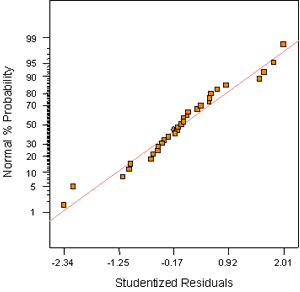Figure B-21. Factorial experiment: normal probability plot for 28-day strength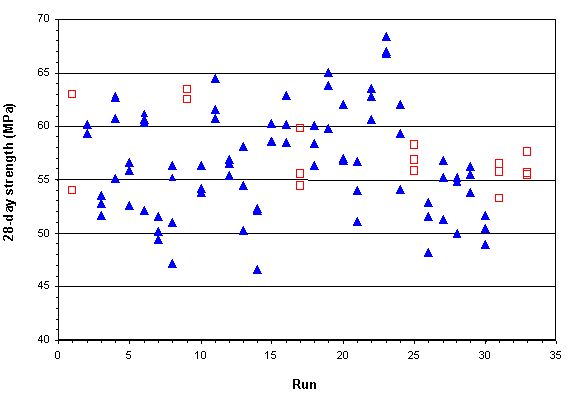Figure B-22. Factorial experiment: raw data plot for 28-day strength (hollow squares indicate control runs)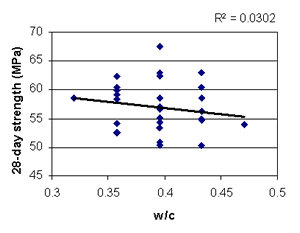Figure B-23. Factorial experiment: scatterplot of 28-day strength vs. w/c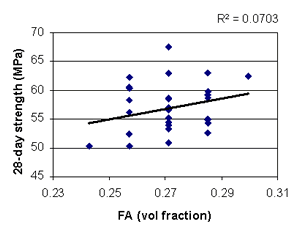Figure B-24. Factorial experiment: scatterplot of 28-day strength vs. fine aggregate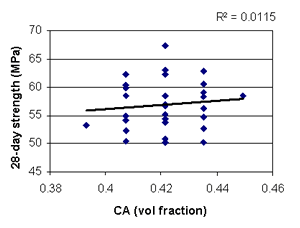Figure B-25. Factorial experiment: scatterplot of 28-day strength vs. coarse aggregate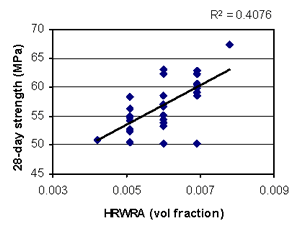Figure B-26. Factorial experiment: scatterplot of 28-day strength vs. HRWRA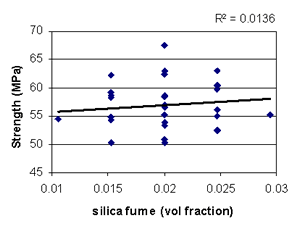Figure B-27. Factorial experiment: scatterplot of 28-day strength vs. silica fume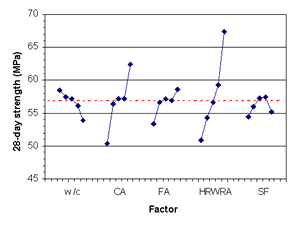FIgure B-28. Factorial experiment: means plot for 28-day strength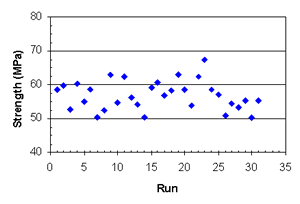Figure B-29. Factorial experiment: 28-day strength vs. run sequence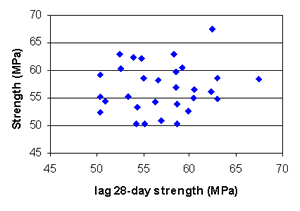Figure B-30. Factorial experiment: lag plot for 28-day strength

Table B-19. Factorial experiment: sequential model sum of squares for RCT charge passed

Source Sum of Squares DF Mean Square F Value Prob > F
Mean315586713155867--
Linear393517.2578703.4327.43< 0.0001
2FI15156.50101515.650.400.9252
Cubic (aliased)10046.8352009.374.770.0557
Residual2104.615420.92--
Total362110331116809.8--

Table B-20. Factorial experiment: lack-of-fit test for RCT charge passed

Source Sum of Squares DF Mean Square F Value Prob > F
Linear69614.70213314.996.300.0432
2FI54458.20114950.759.410.0221
Cubic (aliased)0.6110.61.0010.9745
Pure error2104.004526.00--

Table B-21. Factorial experiment: ANOVA for RCT charge passed model

Source Sum of Squares DF Mean Square F Value Prob > F
Model441455.3 6 73575.89 74.25 < 0.0001
A81666.67 1 81666.67 82.42 < 0.0001
B6868.17 1 6868.17 6.93 0.0146
C11440.67 1 11440.67 11.55 0.0024
E292604.2 1 292604.2 295.30 < 0.0001
E238369.41 1 38369.41 38.72 < 0.0001
AE10506.25 1 10506.25 10.60 0.0034
Residual23780.54 24 990.86 - -
Lack of fit21676.54 20 1083.83 2.06 0.2537
Pure error2104.00 4 526.00 - -
Cor total465235.9 30 - - -

Table B-22. Factorial experiment: summary statistics for RCT charge passed model

 Std. Dev. 31.48 R-Squared 0.9489 Mean 319.06 Adj R-Squared 0.9361 C.V. 9.87 Pred R-Squared 0.8784 PRESS 56577 Adeq Precision 34.166

Table B-23. Factorial experiment: coefficient estimates for RCT charge passed model

Factor Coefficient Estimate DF Standard Error 95% CI Low 95% CI High
Intercept291.11 1 7.22 276.20 306.01
A (w/c)58.33 1 6.43 45.07 71.59
B (fine agg)-16.92 1 6.43 -30.18 -3.66
C (coarse agg)-21.83 1 6.43 -35.09 -8.57
E (silica fume)-110.42 1 6.43 -123.68 -97.16
E236.11 1 5.80 24.14 48.09
AE-25.63 1 7.87 -41.87 -9.38

Model equation for RCT charge passed in terms of coded factors:

RCT charge passed = 291.11 + 58.33*A - 16.92*B - 21.83*C - 110.42*E + 36.11*E2 - 25.63*A*E

Model equation for RCT charge passed in terms of actual factors:

RCT charge passed = 635.4 + 4445.6*w/c - 1199.8*fine agg - 1548.5*coarse agg - 31651*silica fume + 1.635 x 106*(silica fume)2 - 1.448 x 105*w/c*silica fume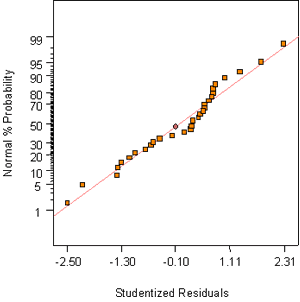Figure B-31. Factorial experiment: normal probability plot for RCT charge passed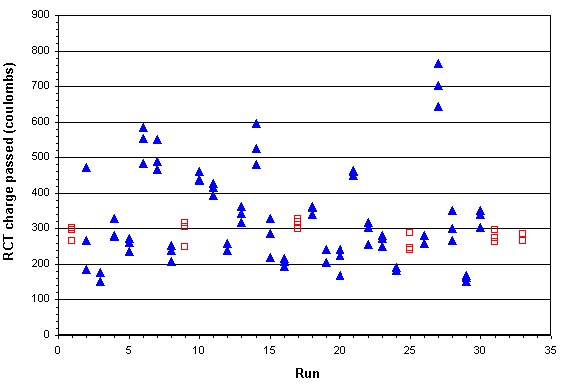Figure B-32. Factorial experiment: raw data plot for RCT charge passed (hollow squares indicate control runs)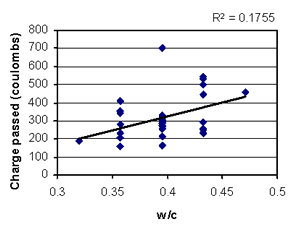Figure B-33. Factorial experiment: scatterplot of RCT charge passed vs. w/c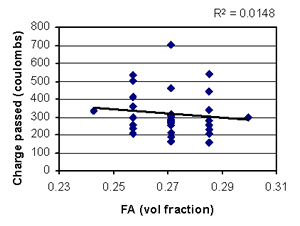Figure B-34. Factorial experiment: scatterplot of RCT charge passed vs. fine aggregate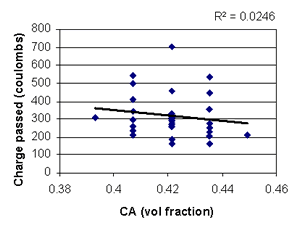Figure B-35. Factorial experiment: scatterplot of RCT charge passed vs. coarse aggregate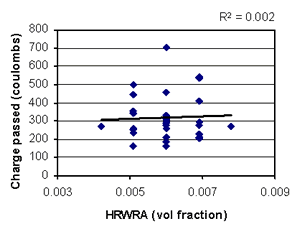Figure B-36. Factorial experiment: scatterplot of RCT charge passed vs. HRWRA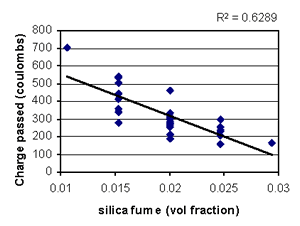Figure B-37. Factorial experiment: scatterplot of RCT charge passed vs. silica fume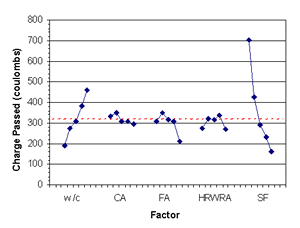Figure B-38. Factorial experiment: means plot for RCT charge passed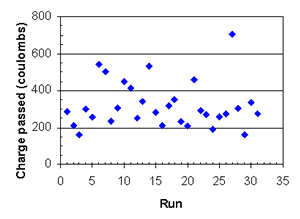Figure B-39. Factorial experiment: RCT charge passed vs. run sequence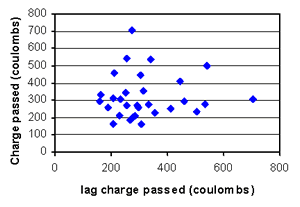Figure B-40. Factorial experiment: lag plot for RCT charge passed